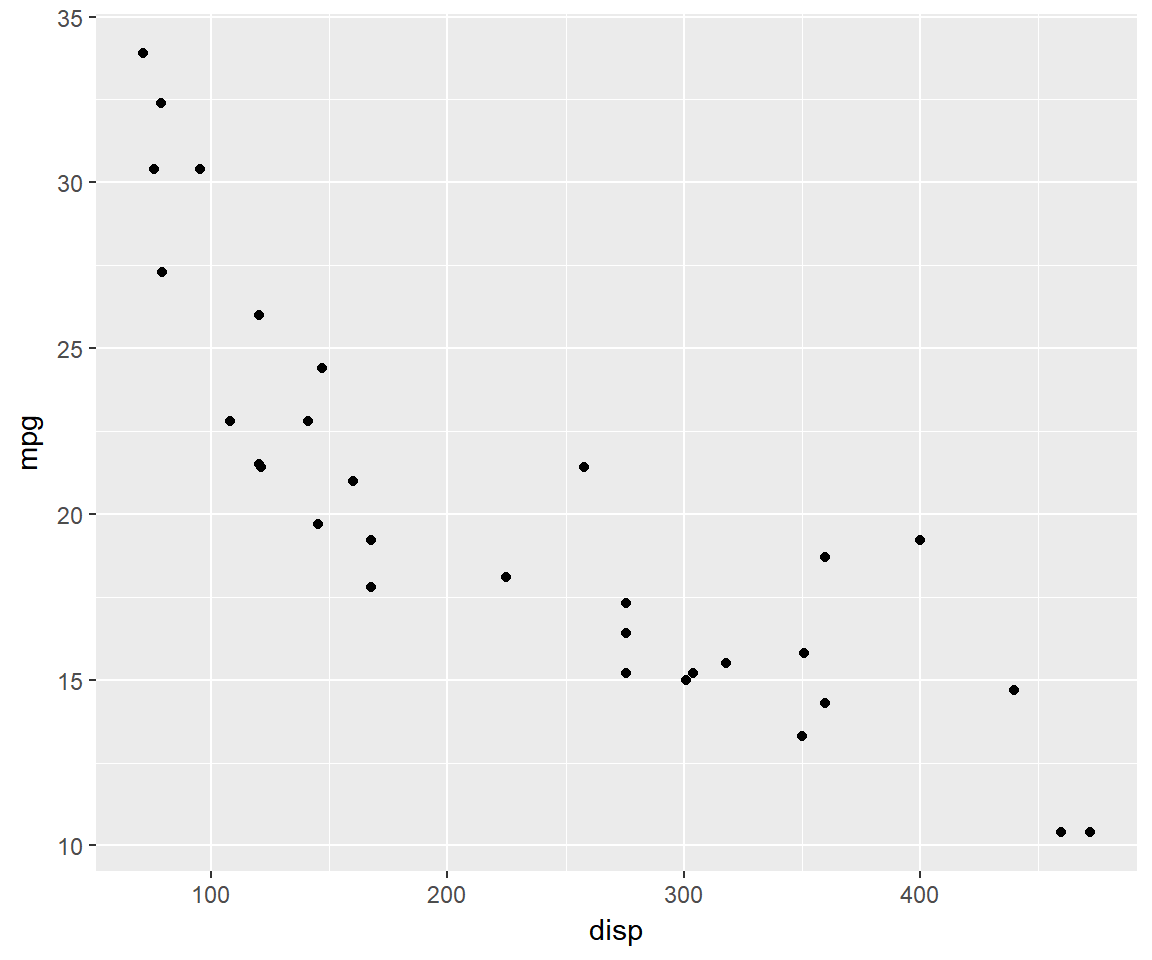## Agenda

Learn to quickly build a set of plots that are routinely used to explore data:

• Scatter Plot
• Bar Plot
• Box Plot
• Line Chart
• Histogram

## Libraries

``````library(ggplot2)
library(dplyr)``````

## qplot

Shortcut designed for those familiar with base plots. You can quickly produce a number of different types of plots. Below are the key arguments:

• `x` : data for X axis
• `y` : data for Y axis
• `geom` : symbols to represent data
• `data` : a data frame or a tibble

## Scatter Plot

``qplot(disp, mpg, data = mtcars)``## Scatter Plot

``qplot(disp, mpg, data = mtcars, geom = c('point', 'line'))``